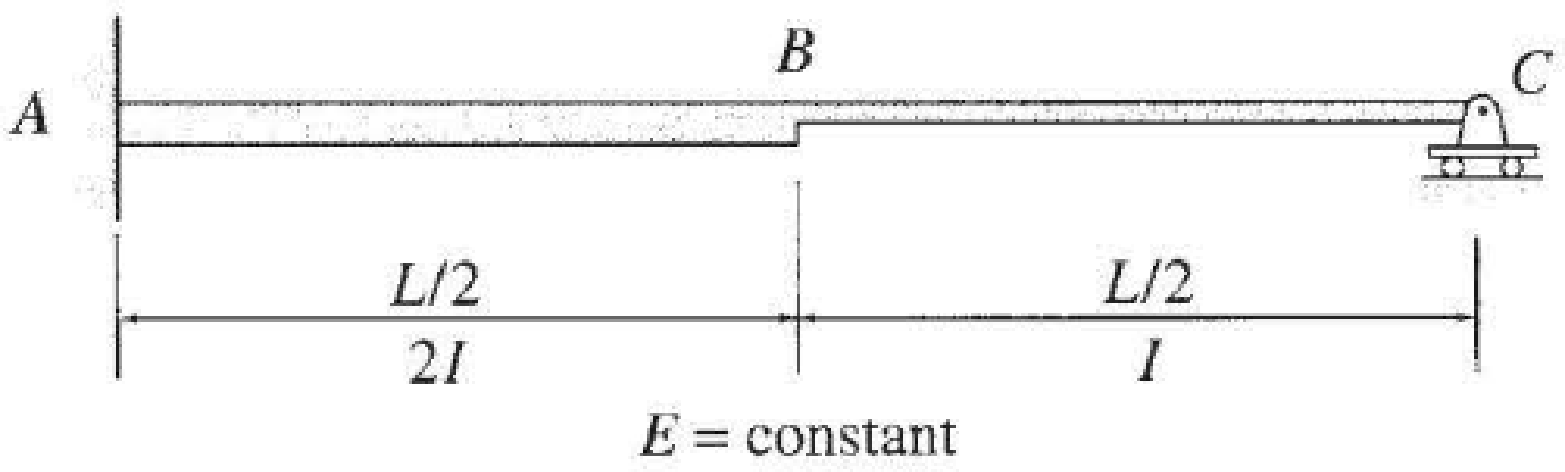# Determine the reactions for the beam shown in Fig. P13.48 due to a small settlement Δ at the roller support C . FIG. P13.48

#### Solutions

Chapter
Section
Chapter 13, Problem 48P
Textbook Problem
10 views

## Determine the reactions for the beam shown in Fig. P13.48 due to a small settlement Δ at the roller support C.FIG. P13.48To determine

Calculate the reactions at the supports due to the small settlement at C for the given beam.

### Explanation of Solution

Given information:

The settlement at support C is Δ.

Apply the sign conventions for calculating reactions, forces, and moments using the three equations of equilibrium as shown below.

• For summation of forces along x-direction is equal to zero (Fx=0), consider the forces acting towards right side as positive (+) and the forces acting towards left side as negative ().
• For summation of forces along y-direction is equal to zero (Fy=0), consider the upward force as positive (+) and the downward force as negative ().
• For summation of moment about a point is equal to zero (Matapoint=0), consider the clockwise moment as negative and the counter clockwise moment as positive.

Calculation:

Show the free body diagram of the given beam as shown in Figure 1.

Refer Figure 1.

Consider the horizontal and vertical reaction at A are denoted by Ax and Ay.

Consider the moment at A is denoted by MA.

Consider the vertical reaction at C is denoted by Cy.

The reactions acting in the beam is 4.

The number of Equilibrium reaction is 3. Then,

The degree of indeterminacy of the beam is 1.

Take the vertical reaction at C as the redundant.

The deflection at C due to the external moment is zero.

Modify the Figure 1 as shown in Figure 2.

Refer Figure 2.

The deflection coefficient representing the deflection at C due to unit value of redundant Cy is fCC.

The deflection at C due to unknown redundant Cy is fCCCy.

Determine the bending moment at x for segment CB.

Mx=1×x=x

Determine the bending moment at x for segment BA.

Mx=1×x=x

Calculate the value of fCC using the virtual work method as shown below.

fCC=(Mx)2EIdx

Substitute x for Mx for the span CB and x for Mx and 2I for I for the span BA.

fCC=0L2(x)2EIdx+L2L(x)22EIdx=1EI(0L2x2dx+12L2Lx2dx)=1EI[(x33)0L2+12(x33)L2L]=1EI[((L2)330)+12(L33(L2)33)

### Still sussing out bartleby?

Check out a sample textbook solution.

See a sample solution

#### The Solution to Your Study Problems

Bartleby provides explanations to thousands of textbook problems written by our experts, many with advanced degrees!

Get Started

Find more solutions based on key concepts
Use A992 steal and. select a W shape. a. Use LRFD. b. Use ASD.

Steel Design (Activate Learning with these NEW titles from Engineering!)

Present Example 13.1 in Chapter 13 using the format discussed in Section 4.2. Divide the example problem into G...

Engineering Fundamentals: An Introduction to Engineering (MindTap Course List)

What is an advantage of using locking pliers?

Precision Machining Technology (MindTap Course List)

Should the IT director report to the company president, or somewhere else? Does it matter?

Systems Analysis and Design (Shelly Cashman Series) (MindTap Course List)

Why do many organizations ban port scanning activities on their internal networks?

Principles of Information Security (MindTap Course List)

List guidelines for conducting NFC transactions safely.

Enhanced Discovering Computers 2017 (Shelly Cashman Series) (MindTap Course List)

If your motherboard supports ECC DDR3 memory, can you substitute non-ECC DDR3 memory?

A+ Guide to Hardware (Standalone Book) (MindTap Course List)

List some applications that use low-pressure piping.

Welding: Principles and Applications (MindTap Course List)